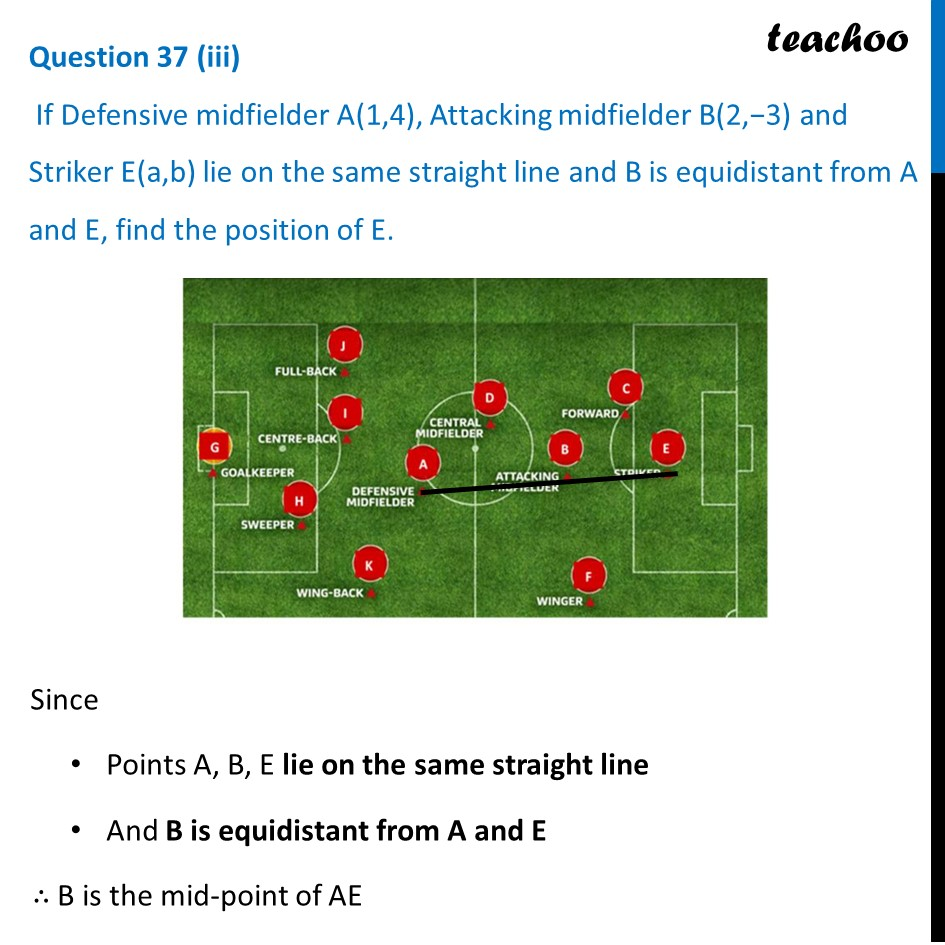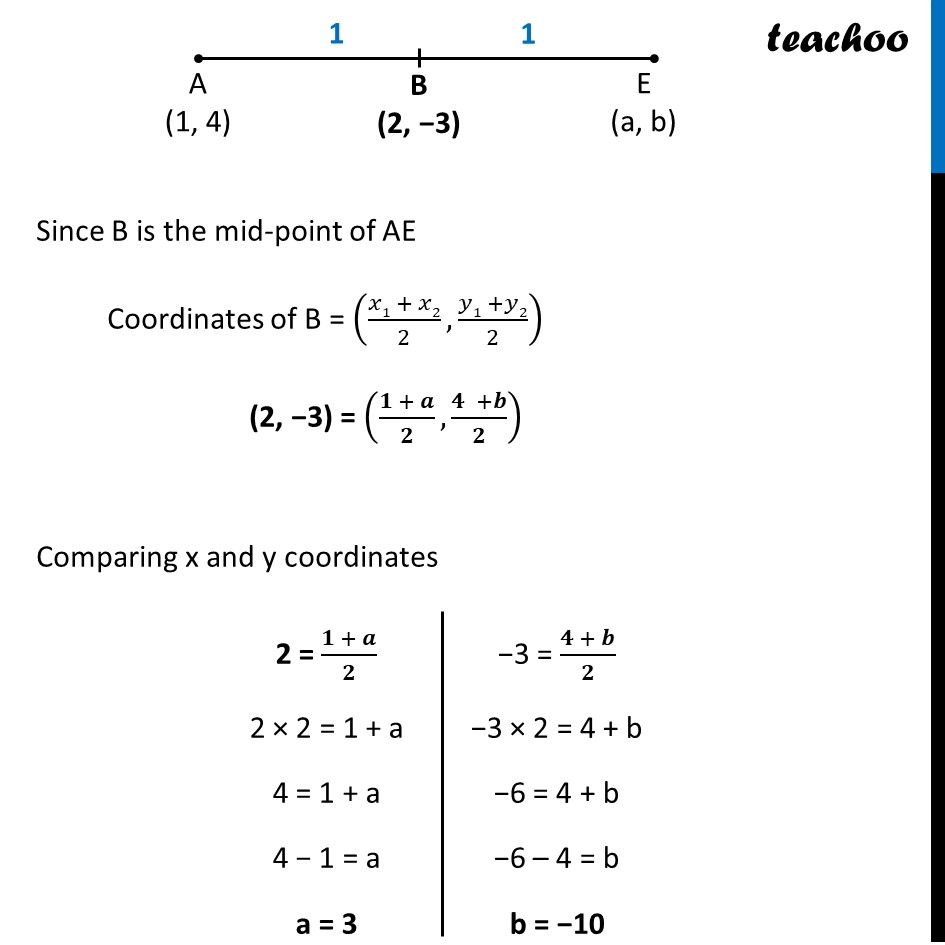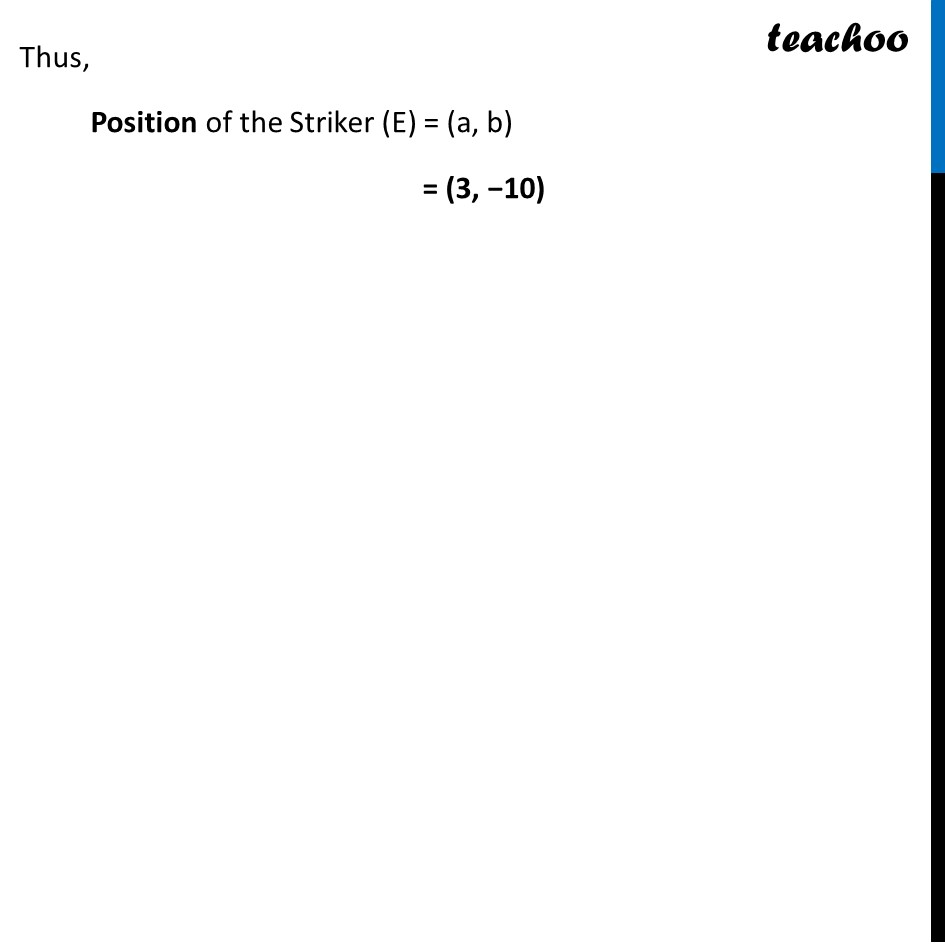CBSE Class 10 Sample Paper for 2024 Boards - Maths Standard

Class 10
Solutions of Sample Papers for Class 10 Boards

## If Defensive midfielder A(1,4), Attacking midfielder B(2,−3) and Striker E(a,b) lie on the same straight line and B is equidistant from A and E, find the position of E.Learn in your speed, with individual attention - Teachoo Maths 1-on-1 Class

### Transcript

Since Points A, B, E lie on the same straight line And B is equidistant from A and E ∴ B is the mid-point of AE Since B is the mid-point of AE Coordinates of B = ((𝑥1 + 𝑥2)/2,(𝑦1 +𝑦2)/2) (2, −3) = ((𝟏 + 𝒂)/𝟐,(𝟒 +𝒃)/𝟐) Comparing x and y coordinates 2 = (𝟏 + 𝒂)/𝟐 2 × 2 = 1 + a 4 = 1 + a 4 − 1 = a a = 3 −3 = (𝟒 + 𝒃)/𝟐 −3 × 2 = 4 + b −6 = 4 + b −6 – 4 = b b = −10 Thus, Position of the Striker (E) = (a, b) = (3, −10)